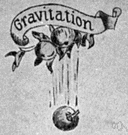gravitational force

(redirected from Newton's law of universal gravitation)
Also found in: Thesaurus, Encyclopedia, Wikipedia.

gravitational force

n.
The weakest of the four fundamental forces of nature, being the attractive force that arises from gravitational interaction. Newton's law of gravity states that the gravitational force between two bodies is proportional to the product of their masses and inversely proportional to the square of the distance between them.
ThesaurusAntonymsRelated WordsSynonymsLegend:
 Noun 1gravitational force - (physics) the force of attraction between all masses in the universe; especially the attraction of the earth's mass for bodies near its surface; "the more remote the body the less the gravity"; "the gravitation between two bodies is proportional to the product of their masses and inversely proportional to the square of the distance between them"; "gravitation cannot be held responsible for people falling in love"--Albert Einsteinnatural philosophy, physics - the science of matter and energy and their interactions; "his favorite subject was physics"attraction, attractive force - the force by which one object attracts anothersolar gravity - the gravity of the sun; "solar gravity creates extreme pressures and temperatures"
References in periodicals archive ?
Both postulates are essential in Newton's law of universal gravitation and in Einstein's general theory of relativity.
They had not, however, yet formally encountered Newton's law of universal gravitation. The students were familiar with the idea of representing vector quantities (such as forces) diagrammatically using arrows, as was required in the questionnaire.

Site: Follow: Share:
Open / Close